# find ∇f at the given point. ƒ(x, y, z) = (x2 + y2 + z2)^(-1/2) + ln (xyz), (-1, 2, -2)

Question
1 views

find ∇f at the given point. ƒ(x, y, z) = (x2 + y2 + z2)^(-1/2) + ln (xyz), (-1, 2, -2)

check_circle

Step 1

Given function is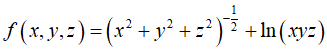Firstly, we find all first order partial derivatives of f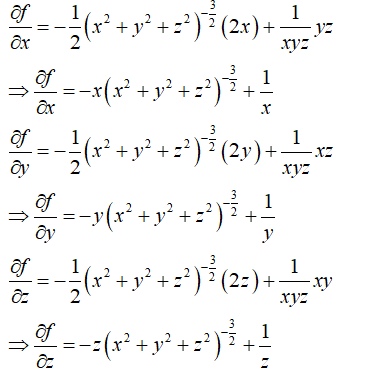Step 2

Now, we find the value of these partial derivatives of f at point (-1,2,-2)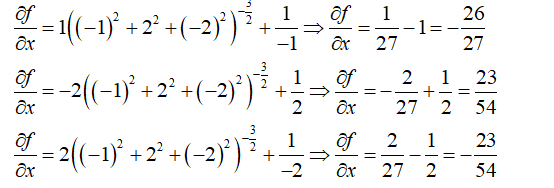Step 3

We know that gradient of f is defined as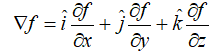Now, at (-1,2,-2), gradient of f is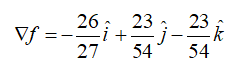...

### Want to see the full answer?

See Solution

#### Want to see this answer and more?

Solutions are written by subject experts who are available 24/7. Questions are typically answered within 1 hour.*

See Solution
*Response times may vary by subject and question.
Tagged in